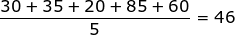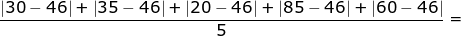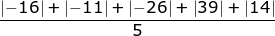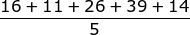# Measures of Variability : Mean Absolute Deviation

The mean absolute value deviation is a measure of dispersion that gives the average variation of the data from the mean.

Example: find the mean absolute value deviation from the given data:

30, 35, 20, 85, 60Where x1is the first term and x2is the second term and so on....is the mean average:n is the number of terms in the data = 5

Now let's substitute the information into the formula:==

21.2 mean absolute deviation

Summarize: to find the mean absolute value deviation you will need to find the average of the data, the number of terms and then substitute that information in to the formula and simplify.

 Related Links: Math algebra Measures of Variability : Interquartile Range Measures of Variability : Range

To link to this Measures of Variability : Mean Absolute Deviation page, copy the following code to your site: